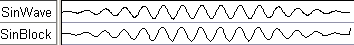## (TD) 4.6 Generating Sine Waves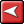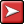## (TD) 4.6 Generating Sine WavesBoth Waveform Block Equations and Label Equations can be used to generate Sine wave signals. There are three functions SinStart, Sin, and SinEnd that generate sine waves with a growing, steady, or decreasing amplitude. Both methods produce waveforms that look the same. However, Waveform Block Equations can be edited to tweak what the waveform looks like. With State Label Equations, each generated state is a separate event and must be individually edited or erased if changes are needed.

SinStart function with State Label Equations

First we will start with the State Label equations where each function has parameters for amplitude, period and duration. There is also an optional fourth parameter that can specify the number of points to use when drawing the waveform. It is not necessary to memorize the syntax of the function, because the equation fly-out will tell you the parameters and function names. The SinStart functions starts a Sine wave with amplitude of 0 and grows to the amplitude specified over the duration specified.

 •Add a new signal and double click on it to open the Signal Properties dialog. Name the signal SinWave, then delete any text that might be in the Label Eqn box. Adjust the placement  of the dialog so that you can see the waveform area and the dialog at the same time. •Next click on the Label Eqn fly out button (see below) and choose SinStart from the list of available functions.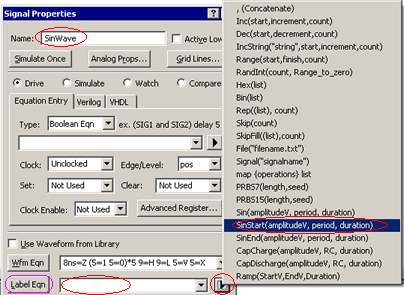•Notice that the SinStart function has been added to the Label Eqn box.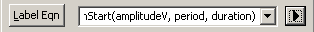•Edit the parameters so that signal will have amplitude of 5, a 20ns period and has reached its full amplitude by 100ns (assuming that ns is the display time unit)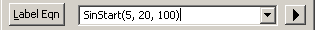•Press the Label Eqn button to generate the start of a Sine wave. The timing diagram editor  will automatically change the radix of the signal to real, because the generator uses floating point numbers to model the waveform. The waveform should look similar to this: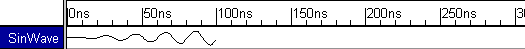Sin function with State Label Equation

The Sin function draws a continuous Sine wave using the specified parameters. Here, we will append to the starting Sine wave drawn on the SinWave signal.

 •Enter Sin(5, 20, 100) in the Label Eqn box either by typing or using the equation flyout.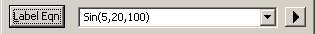•Press the Label Eqn button to continue the Sine wave generation.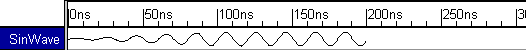Notice that by using the same parameters, the generated Sine wave matches the Start Sine wave that was drawn.

SinEnd function

The SinEnd function does the inverse of the SinStart function. It starts with a Sine wave of the full  amplitude and diminishes over the specified duration until the amplitude is zero.

 •Enter SinEnd(5, 20, 100) in the Label Eqn history either by typing or using the equation flyout.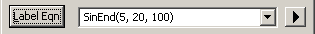•Press the Label Eqn button to finish our Sine wave generation.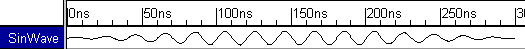Sine waves with Waveform Block Equations

Waveform Equation Blocks preserve the beginning and ending edges of the block so that the block can be dragged and dropped independently of other blocks on the same signal. In the picture below, the waveform was created by inserting waveform equation blocks into consecutive valid segments. This caused unsightly black lines to be drawn between the segments.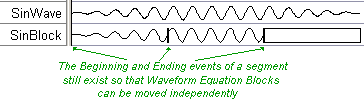If you delete the black lines between two waveform equation blocks, then the blocks are said to be chained together. Each block can still be edited, but the starting time of the second block is now fixed at the ending time of the first block. A quick way to add chained blocks is to add a block to the middle of a long waveform segment:

 •Add a new signal and double click on it to open the Signal Properties dialog. Name the signal SinBlock.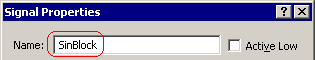•Draw one long segment on the SinBlock signal that is at least 250ns long.•Double click on the segment to open the Edit Bus State dialog. •Press the Insert Block button to open the Waveform Equation Block Properties dialog.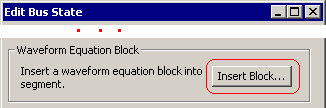•Choose Sine Wave Start from the flyout.•Change Duration to 100 ns.•Edit the code so that period_ns = 20.0•Press the OK button to close the dialog and draw the waveform. Notice that there is not a black line at the end.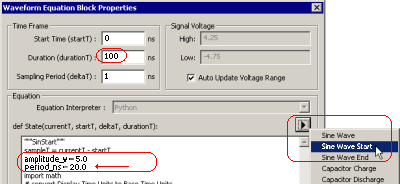•Double click on rest of the segment to open the Waveform Equation Block Properties dialog.•Choose Sine Wave from the flyout.•Change Duration to 100 ns.•Edit the code so that period_ns = 20.0•Press the OK button to draw the waveform.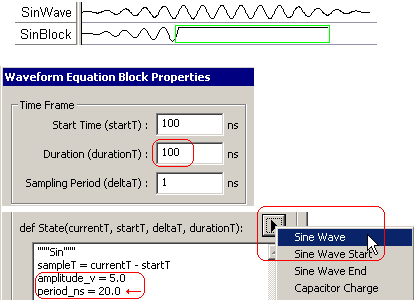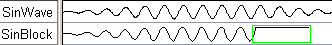•Add the sine wave ending section by choosing Sine Wave End from the flyout and changing Duration to 100ns and setting the period_ns to 20.•There is a small black line at the end of the waveform because this is the event that got moved from 250ns. You can delete it if desired.